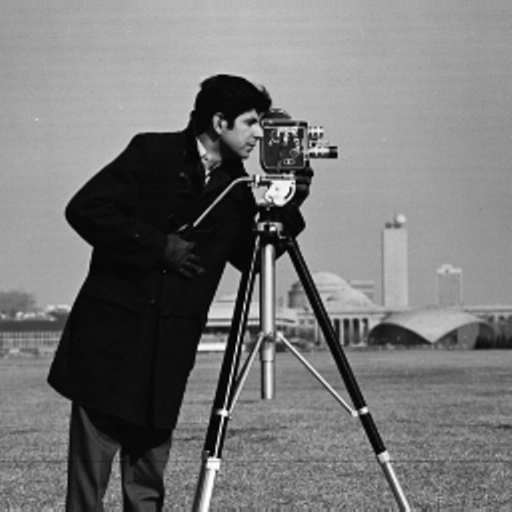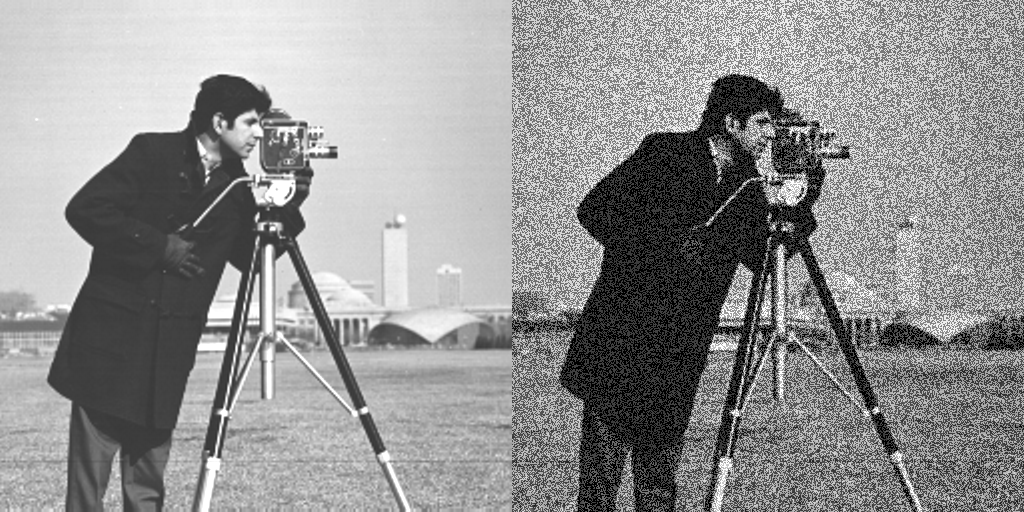# Structural Similarity IndexWhen comparing images, the Mean Squared Error (or MSE), though straightforward to calculate, may not be a very good indicator of their perceived similarity.

The Structural Similarity Index (or SSIM) aims to address this shortcoming by taking texture into account, and assigning a higher score to images that may appear similar.

using Images, TestImages
using Random

img_orig = float64.(testimage("cameraman"))We use a grayscale image out of the TestImages package, which provides a standard suite of test images. float/float32/float64 preserve colorant information: thus the image is now composed of pixels of type Gray{Float64}.

assess_ssim(img_orig, img_orig)
1.0

The assess_ssim function, which takes two images as inputs and returns their structural similarity index, is the simplest way to calculate the SSIM of two images.

An SSIM score of 1.00 indicates perfect structural similarity, as is expected out of identical images.

Now, we create two variations of the original image: image_const on the left has the intensity of all its pixels increased by 0.2 times the intensity range, while image_noise on the right has the intensity of some of its pixels increased, and that of the others decreased by the same amount. The two images look quite different visually.

noise = ones(size(img_orig)) .* 0.2 .* (maximum(img_orig) - minimum(img_orig))
img_const = img_orig + noise

mask = rand(Float64, size(img_orig)) .< 0.5
img_noise = img_orig + noise

mosaicview(img_const, img_noise; nrow=1)We use the mse funtion defined in ImageDistances to calculate the mean squared error between the original and the two modified images.

mse(img_orig, img_const), mse(img_orig, img_noise)
(0.039999999999999016, 0.039999999999999016)

Despite their visual differences, both the images have the exact same mean squared error of 0.400, when compared with the original. This demonstrates how in certain cases, MSE can fail to capture the perceived similarity of images.

assess_ssim(img_orig, img_const), assess_ssim(img_orig, img_noise)
(0.8406360281731596, 0.10496952500790142)

Their SSIM scores vary significantly, with image_const being rated much closer to the original image in terms of perceived similarity, which is in line with what visually seems to be the case.

### Custom Parameters

While assess_ssim is a convenient way to calculate the SSIM of two images, it does not allow for custom parameters to be passed to the SSIM algorithm, for which we have the following syntax.

iqi = SSIM(KernelFactors.gaussian(2.0, 11), (0.5, 0.5, 0.5))
assess(iqi, img_orig, img_const)
0.9085673404877024

Here, the first parameter is the kernel used to weight the neighbourhood of each pixel while calculating the SSIM locally, and defaults to KernelFactors.gaussian(1.5, 11). The second parameter is the set of weights (α, β, γ) given to the lunimance (L), contrast (C) and structure (S) terms while calculating the SSIM, and defaults to (1.0, 1.0, 1.0). Recall that SSIM is defined as Lᵅ × Cᵝ × Sᵞ.

1. Zhou Wang; Bovik, A.C.; ,”Mean squared error: Love it or leave it? A new look at Signal Fidelity Measures,” Signal Processing Magazine, IEEE, vol. 26, no. 1, pp. 98-117, Jan. 2009.
2. Z. Wang, A. C. Bovik, H. R. Sheikh and E. P. Simoncelli, “Image quality assessment: From error visibility to structural similarity,” IEEE Transactions on Image Processing, vol. 13, no. 4, pp. 600-612, Apr. 2004.